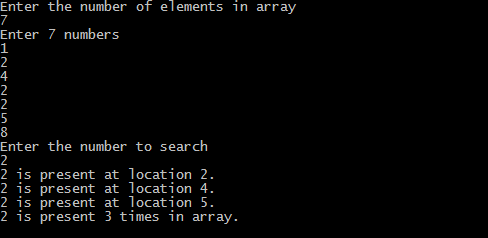## Print this Job Post# Linear search in c

The following code implements linear search (Searching algorithm) which is used to find whether a given number is present in an array and if it is present then at what location it occurs. It is also known as sequential search. It is very simple and works as follows: We keep on comparing each element with the element to search until the desired element is found or list ends. Linear search in c language for multiple occurrences and using function.

## Linear search c program

#include <stdio.h>
#include<conio.h>
void main()
{
int array, search, c, n;
printf("Enter the number of elements in array\n");
scanf("%d",&n);
printf("Enter %d integer(s)\n", n);
for (c = 0; c < n; c++)
scanf("%d", &array[c]);
printf("Enter the number to search\n");
scanf("%d", &search);
for (c = 0; c < n; c++)
{
if (array[c] == search)     /* if required element found */
{
printf("%d is present at location %d.\n", search, c+1);
break;
}
}
if (c == n)
printf("%d is not present in array.\n", search);
getch();
}

Output of program:Linear search for multiple occurrences
In the code below we will print all the locations at which required element is found and also the number of times it occur in the list.
#include <stdio.h>
#include<conio.h>
void main()
{
int array, search, c, n, count = 0;
clrscr();
printf("Enter the number of elements in array\n");
scanf("%d",&n);
printf("Enter %d numbers\n", n);
for ( c = 0 ; c < n ; c++ )
scanf("%d",&array[c]);
printf("Enter the number to search\n");
scanf("%d",&search);
for ( c = 0 ; c < n ; c++ )
{
if ( array[c] == search )
{
printf("%d is present at location %d.\n", search, c+1);
count++;
}
}
if ( count == 0 )
printf("%d is not present in array.\n", search);
else
printf("%d is present %d times in array.\n", search, count);
getch();
}

## Output of the Program:## C program for linear search using function

#include <stdio.h>
#include<conio.h>
int linear_search(int*, int, int);
void main()
{
int array, search, c, n, position;
clrscr();
printf("Enter the number of elements in array\n");
scanf("%d",&n);
printf("Enter %d numbers\n", n);
for ( c = 0 ; c < n ; c++ )
scanf("%d", &array[c]);
printf("Enter the number to search\n");
scanf("%d",&search);
position = linear_search(array, n, search);
if ( position == -1 )
printf("%d is not present in array.\n", search);
else
printf("%d is present at location %d.\n", search, position+1);
getch();
int linear_search(int *pointer, int n, int find)
{
int c;
for ( c = 0 ; c < n ; c++ )
{
if ( *(pointer+c) == find )
return c;
}
return -1;
}
Time required to search an element using linear search algorithm depends on size of list. In best case element is present at beginning of list and in worst case element is present at the end. Time complexity of linear search is O(n).